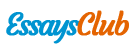# Chemical Reaction Exam

Autor:   •  February 5, 2018  •  1,978 Words (8 Pages)  •  140 Views

Page 1 of 8

...

---------------------------------------------------------------

-

An experiment was conducted as shown in the diagram below.

- [pic 3]

CaCO3(s) was added to HCl(aq). The flask, contents and balloon were weighed before and after the reaction. The products of the reaction are water (H2O), carbon dioxide gas (CO2) and a solution of calcium chloride (CaCl2).

- Write a balanced equation (including states) for the reaction occurring in the flask.

- Would you expect the mass after the reaction to be greater than, less than or equal to the mass before reaction? Justify your choice.

-

-

-

-

-

-

-

-

-

- CaCO3(s) + 2HCl(aq) → CaCl2(aq) + CO2(g) + H2O

- The mass should be the same before and after the reaction. The Law of Conservation of Mass states that mass is neither created nor destroyed during a chemical reaction. orThe mass would be slightly less because some gas might have been lost when the balloon was being attached to the flask, as the reaction was beginning.

5

-

Determine the balanced formula equation, including states, for each of the reactions described below:

- _P4 + _O2 → _P2O5(s)

- _KClO3(s) → _KCl(s) + _O2(g)

- P4 + 5O2 → 2P2O5(s)

- 2KClO3(s) → 2KCl(s) + 3O2(g)

-

6

-

Write a balanced formula equation, including states, for each of the reactions described below.

- When aqueous solutions of lead(II) nitrate, Pb(NO3)2, and potassium iodide, KI, are mixed, the yellow solid lead(II) iodide, PbI2, precipitates from the solution, while the soluble salt, potassium nitrate, KNO3, remains in solution.

- An aqueous solution of ethanol, C2H5OH, is produced when a glucose, C6H12O6, solution reacts in the presence of a yeast catalyst. Gaseous carbon dioxide, CO2, is also produced.

-

-

-

- Pb(NO3)2(aq) + 2KI(aq) → PbI2(s) + 2KNO3(aq)

- C6H12O6(aq) → 2C2H5OH(aq) + 2CO2(g)

-

8

-

Use any of the terms in the list below to identify each of the reactions shown.

decomposition, precipitation, metal displacement, combustion, combination

a Pb(NO3)2(aq) + 2KCl(aq) → PbCl2(s) + 2KNO3(aq)

b C6H12O6(aq) + 6O2(g) → 6CO2(g) + 6H2O(1)

c Mg(s) + ZnCl2(aq) → MgCl2(aq) + Zn(s)

a precipitation

b redox, oxidation or combustion

c redox or metal displacement

3

-

The table below shows the symbol or formula of several ions.

Name of ion

Symbol or formula

ammonium

NH[pic 4]

magnesium

Mg2+

calcium

Ca2+

aluminium

Al3+

carbonate

CO[pic 5]

hydroxide

OH–

nitride

N3–

Construct the chemical formula for:

a magnesium nitride

b ammonium hydroxide

c magnesium hydroxide.

a Mg3N2

b NH4OH

c Mg(OH)2

4

-

Use the information from question 5 to name these chemicals.

a Al2(SO4)3

b MgCO3

c Ca(OH)2

a aluminium sulfate

b magnesium carbonate

c calcium hydroxide

3

-

The table below shows the solubility of several ions.

Negative ion

Positive ion

Solubility of compounds

NO[pic 6]

all

soluble

SO[pic 7]

Ba2+ Pb2+

others

insoluble

soluble

Cl –

...